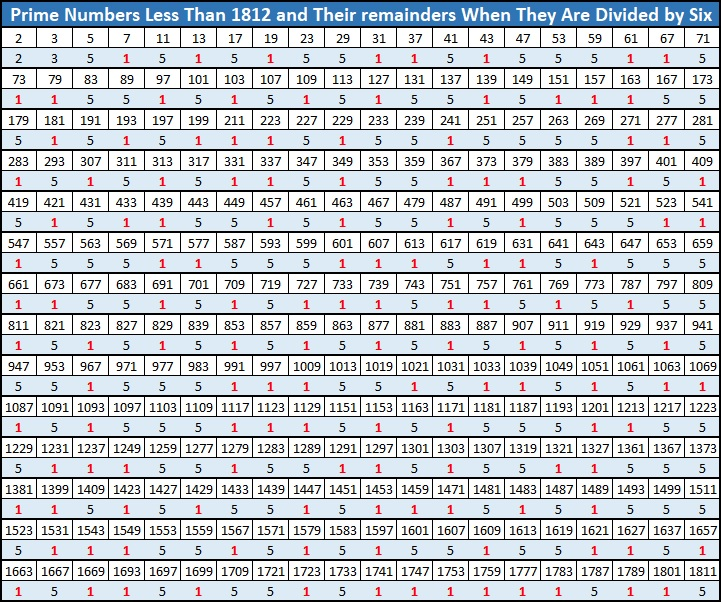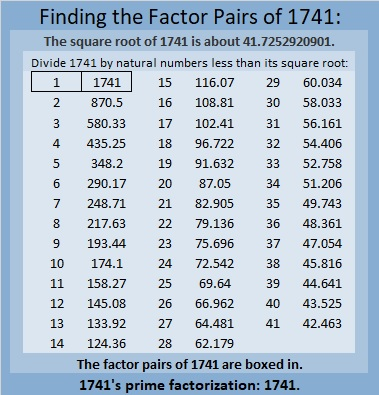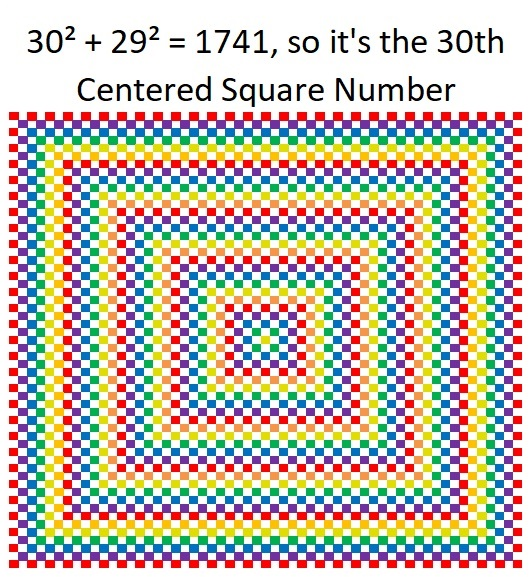# 1741 What Do You Notice About the Remainders?

Contents

### Today’s Puzzle:

The table below is a list of the first 280 prime numbers. Below each prime number is its remainder when the prime number is divided by 6. What do you notice about the remainders? What do you wonder?Did you notice a fun fact starting with the number 1741? I would like to thank OEIS.org for making me aware of it.

### Factors of 1741:

• 1741 is a prime number.
• Prime factorization: 1741 is prime.
• 1741 has no exponents greater than 1 in its prime factorization, so √1741 cannot be simplified.
• The exponent in the prime factorization is 1. Adding one to that exponent we get (1 + 1) = 2. Therefore 1741 has exactly 2 factors.
• The factors of 1741 are outlined with their factor pair partners in the graphic below.How do we know that 1741 is a prime number? If 1741 were not a prime number, then it would be divisible by at least one prime number less than or equal to √1741. Since 1741 cannot be divided evenly by 2, 3, 5, 7, 11, 13, 17, 19, 23, 29, 31, 37, or 41, we know that 1741 is a prime number.

### More About the Number 1741:

1741 is the sum of two squares:
30² + 29² = 1741.

Here’s another way we know that 1741 is a prime number: Since its last two digits divided by 4 leave a remainder of 1, and 30² + 29² = 1741 with 30 and 29 having no common prime factors, 1741 will be prime unless it is divisible by a prime number Pythagorean triple hypotenuse less than or equal to √1741. Since 1741 is not divisible by 5, 13, 17, 29, 37, or 41, we know that 1741 is a prime number.

Since 29 and 30 are consecutive numbers, we have another fun fact:And 1741 is the hypotenuse of a Pythagorean triple:
59-1740-1741, calculated from 30² – 29², 2(30)(29), 30² + 29².

1741 is also the difference of two consecutive squares:
871² – 870² = 1741.

This site uses Akismet to reduce spam. Learn how your comment data is processed.